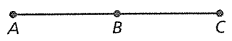Mathematics
Easy

Question

# Four steps of a proof are shown.Given: AC = AB + ABProve: AB = BCWhat is step no. 4? Statements Reason 1. AC = AB + AB 1. Given 2. AB + BC = AC   3. AB + AB = AB + BC   4. AB = BC

## Substitution Property of EqualityTransitive propertySegment Addition PostulateSubtraction property of equalityHint:

## The correct answer is: Subtraction property of equality

### Given AB + BC = AB + ABSo , using Subtraction property of equality remove AB from both side we getBC = AB .Subtraction property of equality  is the right choice .

The property is Subtraction property of equality
The formula we use to tell us this is if a = b, then a - c = b - c

### Related Questions to study#### With Turito Foundation.#### Get an Expert Advice From Turito.